# 无人驾驶汽车系统入门（十六）——最短路径搜索之A*算法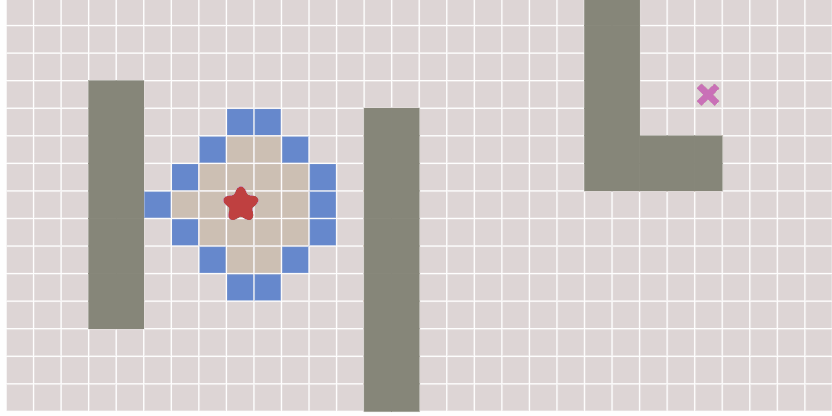BFS代码：

frontier = Queue()
frontier.put(start)
visited = {}
visited[start] = True

while not frontier.empty():
current = frontier.get()
for next in graph.neighbors(current):
if next not in visited:
frontier.put(next)
visited[next] = True

## 所涉及到的主要数据结构:

1. graph，要找到一张图中两点之间的path，我们需要一个最基本的graph数据结构。在本文中，我们只需要得到某一点的邻近点，在这里我们的代码调用graph.neighbors(current)，该函数返回点current周围的所有邻近点构成的一个列表，由for循环可以遍历这个列表

2. queue 为了解释用队列的原因，请看下图，假设此时frontier为空，current当前是A点，它的neighbors将返回B、C、D、E四个点，在将这4个点都添加到frontier当中以后，下一轮while循环，frontier.get()将返回B点（根据FIFO原则，B点最早入队，应当最早出队），此时调用neighbors，返回A、f、g、h四个点，除了A点，其他3个点又被添加到frontier当中去。再到下一轮循环，此时frontier当中有C、D、f、g、h这几个点，由于队列的FIFO原则，frontier.get()将返回C点。这样就保证了整个扩散过程是由近到远，由内而外的，这也是广度优先搜索的原则。可以看到，frontier.get()从队列中取出一个元素（该元素将从队列中被删除）。而frontier.put()将current的邻近点又添加进去，整个过程不断重复，直到图中的所有点都被遍历一遍。

3. visited列表：接着上面的讨论，graph.neighbors(A)将返回B、C、D、E 4个点，随后这4个点被添加到frontier当中，下一轮graph.neighbors(B)将返回A、h、f、g四个点，而加入此时A再被添加到frontier当中就导致遍历陷入死循环，为了避免这种状况出现，我们需要将已经遍历过了的点添加到visited列表当中，之后在将点放入frontier之前，首先判断该点是否已经在visited列表当中。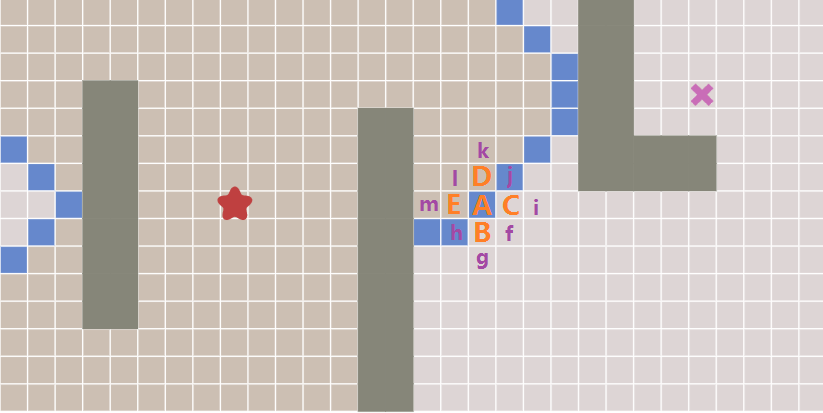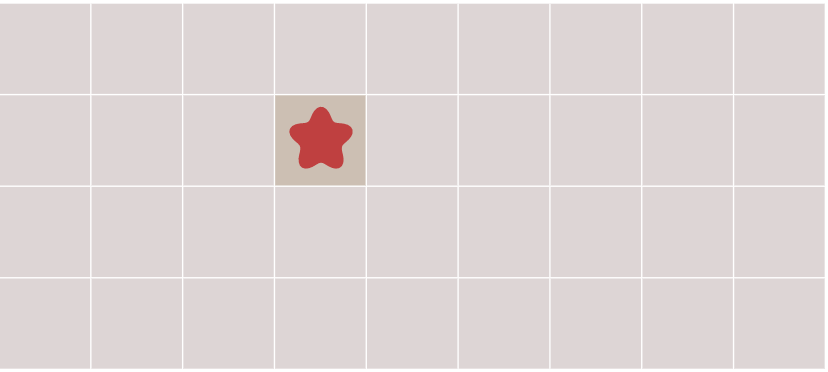## 找到路线

frontier = Queue()
frontier.put()
came_from = {}
came_from[start] = None

while not frontier.empty():
current = frontier.get()
for next in graph.neighbors(current):
if next not in came_from:
frontier.put(next)
came_from[next] = current

current = goal
path = []
while current != start
path.append(current)
current = came_from[current]
path.append(start)
path.reverse()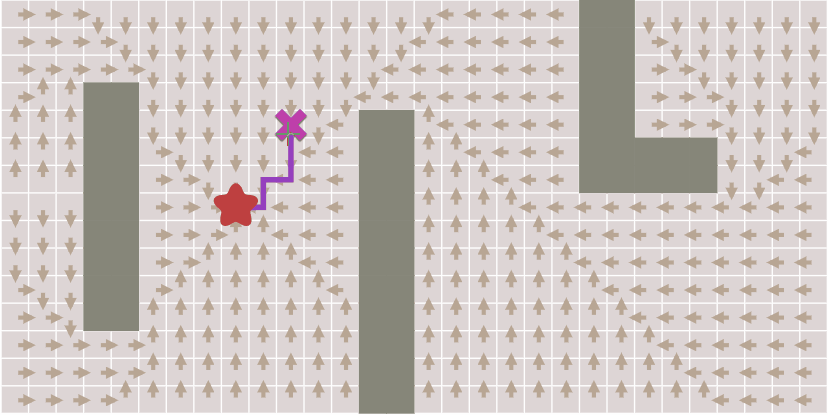### 提前结束

frontier = Queue()
frontier.put(start)
came_from = {}
came_from[start] = None

while not frontier.empty():
current = frontier.get()

if current == goal
break

for next in graph.neighbors(current):
if next not in came_from:
frontier.put(next)
came_from[next] = current


## 扩散的方向性

def heuristic(a, b):
# 这种距离叫做曼哈顿距离（Manhattan）
return abs(a.x - b.x) + abs(a.y - b.y)

## 启发式的搜索

frontier = PriorityQueue()
frontier.put(start, 0)
came_from = {}
came_from[start] = None

while not frontier.empty():
current = frontier.get()

if current == goal:
break

for next in graph.neighbors(current):
if next not in came_from:
priority = heuristic(goal, next)
frontier.put(next, priority)
came_from[next] = current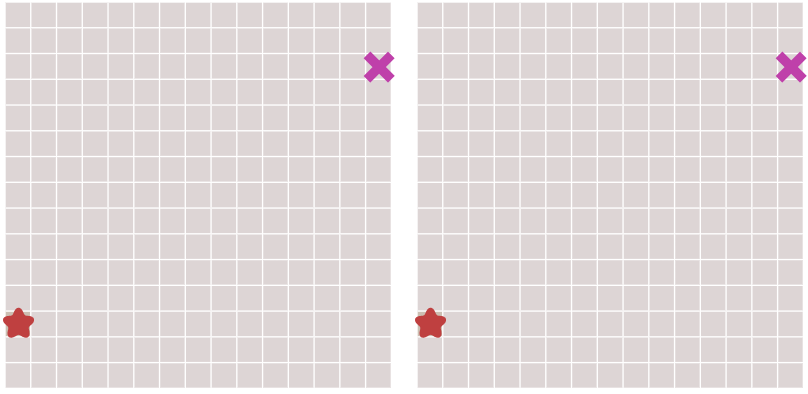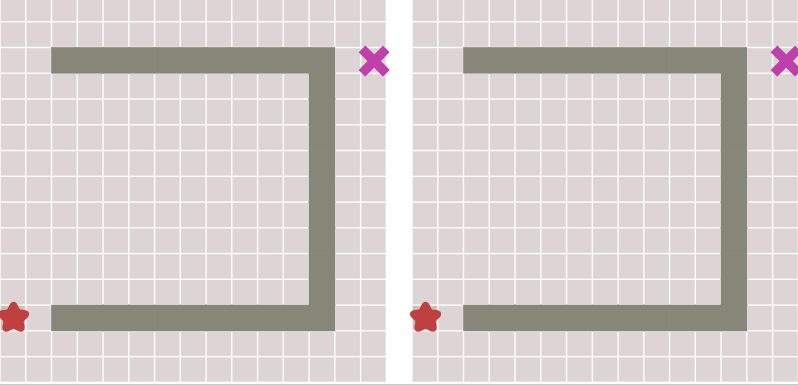## Dijkstra:

frontier = PriorityQueue()
frontier.put(start, 0)
came_from = {}
cost_so_far = {}
came_from[start] = None
cost_so_far[start] = 0

while not frontier.empty():
current = frontier.get()

if current == goal:
break

for next in graph.neighbors(current):
new_cost = cost_so_far[current] + 1
if next not in cost_so_far or new_cost < cost_so_far[next]:
cost_so_far[next] = new_cost
priority = new_cost
frontier.put(next, priority)
came_from[next] = current

## A*算法

frontier = PriorityQueue()
frontier.put(start, 0)
came_from = {}
cost_so_far = {}
came_from[start] = None
cost_so_far[start] = 0

while not frontier.empty():
current = frontier.get()

if current == goal:
break

for next in graph.neighbors(current):
new_cost = cost_so_far[current] + 1
if next not in cost_so_far or new_cost < cost_so_far[next]:
cost_so_far[next] = new_cost
priority = new_cost + heuristic(goal, next)
frontier.put(next, priority)
came_from[next] = current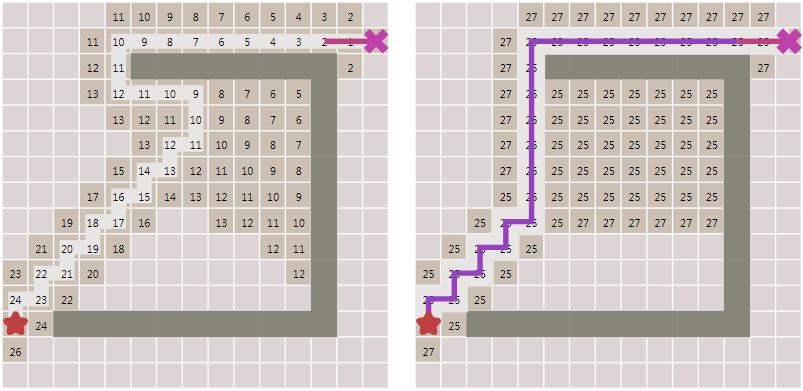priority = new_cost + heuristic(goal, next)

AdamShan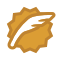CSDN认证博客专家 图像处理 深度学习 TensorFlow

02-18776403-1621万+
11-062万+
06-099950
03-172444
07-141933
09-206079
09-021万+
10-165万+点击重新获取扫码支付余额充值